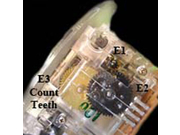# MAN Trucks - All

Individual Odometer Repair PartsMAN Truck VDO Individual Odometer Gears
E1 gear is always the 12 tooth drive gear, E2 is always the second gear 48x12 with flat back, E3 gear has 48 teeth on the outside and then between 12 and 21 teeth on the underside gear, original gear is amber in color. (Must count teeth on original gear before ordering). {If you cannot count the teeth on the main gear then count the teeth of the next gear that it meshes with. If your tooth count is 39=48x21, 41=48x19, 42=48x18, 43=48x17, 44=48x16, 45=48x15, 46=48x14, 47=48x13, 48=48x12}
\$25.00 Select a style:MAN trucks VDO Three Gear Set
There are two tooth count options for this application. E3 gear has 48 teeth on the outside and then either 12, 13, 16, 17 or 18 teeth on the underside gear, original gear is amber in color. (Must count teeth on original gear before ordering).
{If you cannot count the teeth on the main gear then count the teeth of the next gear that it meshes with. If your tooth count is 39=48x21, 41=48x19, 42=48x18, 43=48x17, 44=48x16, 45=48x15, 46=48x14, 47=48x13, 48=48x12}

\$65.00 Select a style: